# CRYSTAL LATTICE UNIT CELL Crystal Lattice a regular

• Slides: 34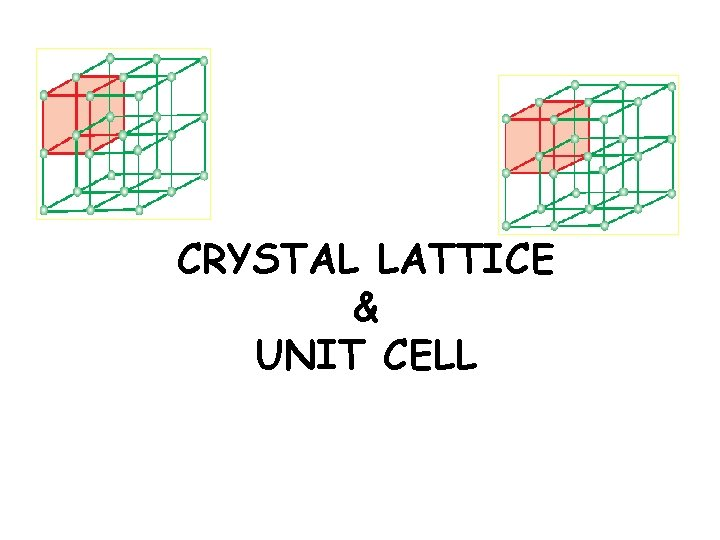CRYSTAL LATTICE & UNIT CELLCrystal Lattice. a regular three dimensional arrangement of points in space is called a crystal lattice.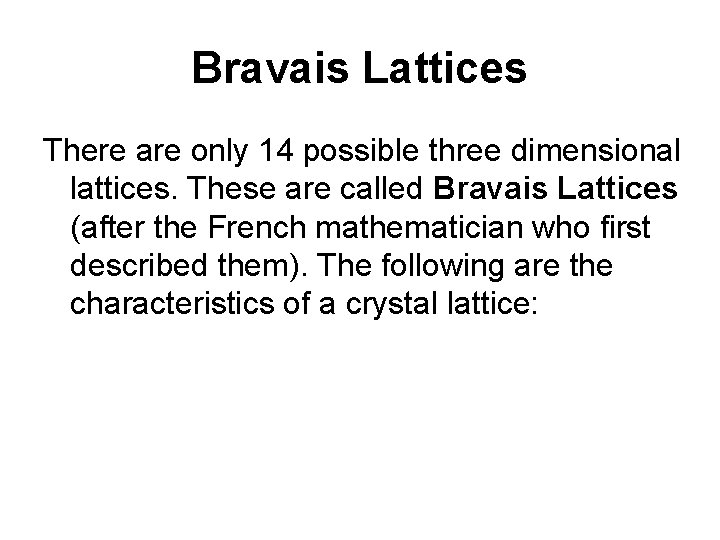Bravais Lattices There are only 14 possible three dimensional lattices. These are called Bravais Lattices (after the French mathematician who first described them). The following are the characteristics of a crystal lattice: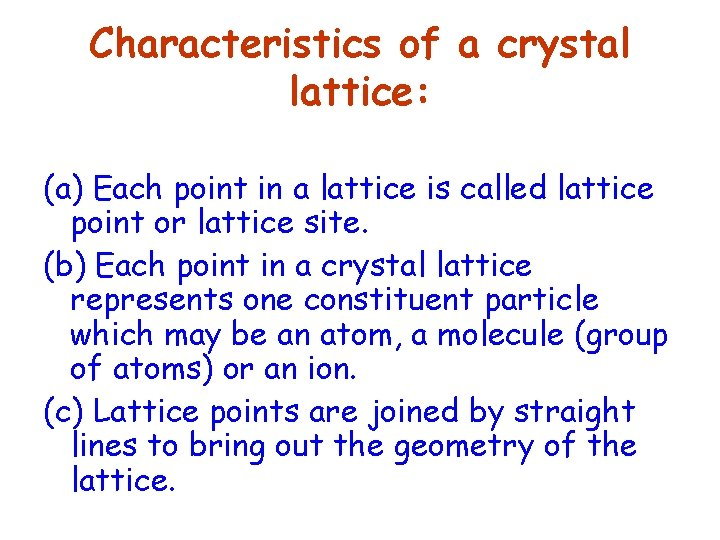Characteristics of a crystal lattice: (a) Each point in a lattice is called lattice point or lattice site. (b) Each point in a crystal lattice represents one constituent particle which may be an atom, a molecule (group of atoms) or an ion. (c) Lattice points are joined by straight lines to bring out the geometry of the lattice.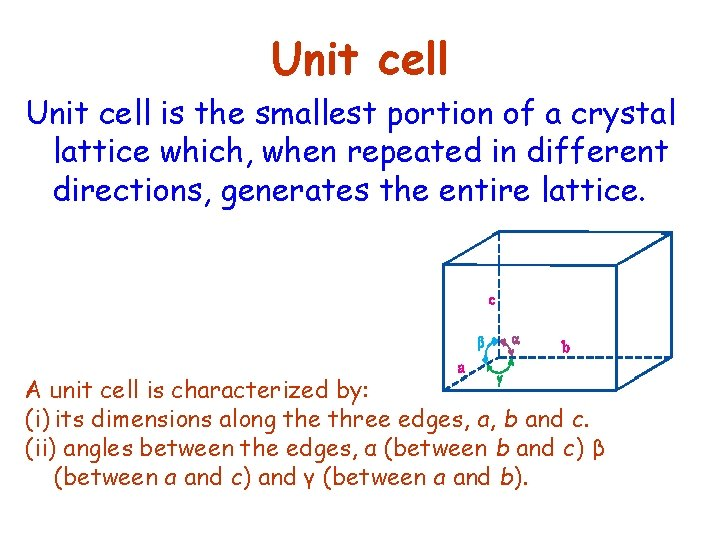Unit cell is the smallest portion of a crystal lattice which, when repeated in different directions, generates the entire lattice. A unit cell is characterized by: (i) its dimensions along the three edges, a, b and c. (ii) angles between the edges, α (between b and c) β (between a and c) and γ (between a and b).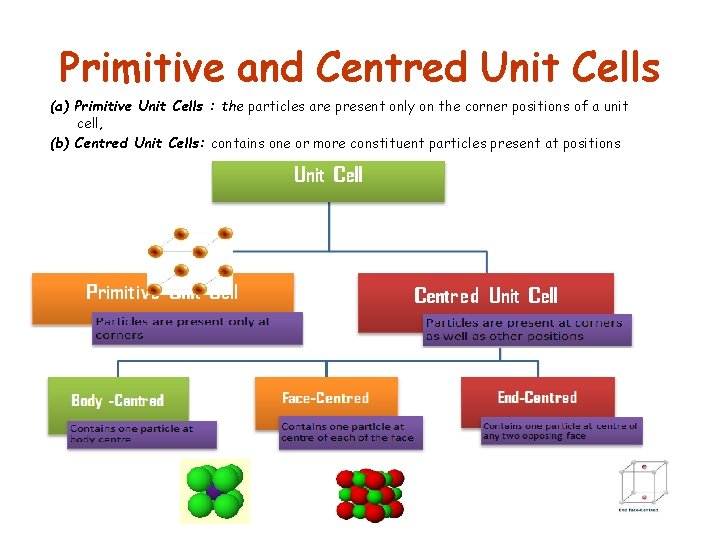Primitive and Centred Unit Cells (a) Primitive Unit Cells : the particles are present only on the corner positions of a unit cell, (b) Centred Unit Cells: contains one or more constituent particles present at positions other than corners in addition to those at corners.The three cubic lattices: all sides of same length, angles between faces all 90°In the following structure , each corner atom is shared between how many unit cells ? each corner atom is shared between 8 unit cellsprimitive cubic unit cell (a) open structure (b) space-filling structure (c) actual portions of atoms belonging to one unit cell. 1/8 atom the total number of atoms in one unit cell = 8 x 1/8 = 1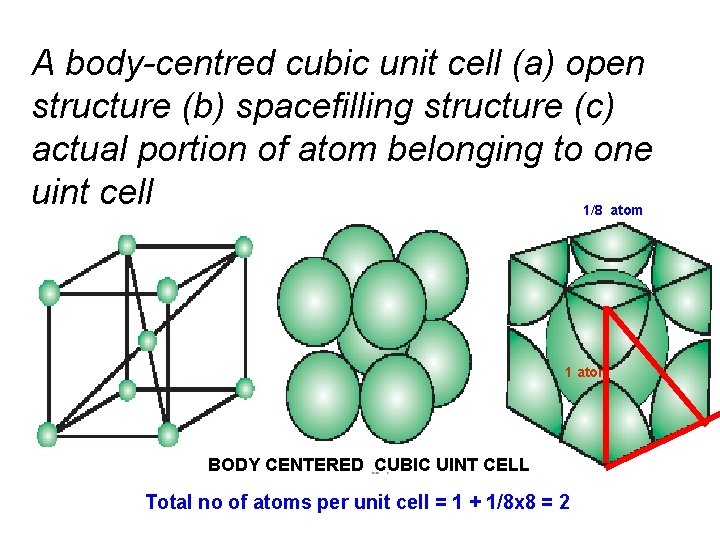A body-centred cubic unit cell (a) open structure (b) spacefilling structure (c) actual portion of atom belonging to one uint cell 1/8 atom 1 atom BODY CENTERED CUBIC UINT CELL Total no of atoms per unit cell = 1 + 1/8 x 8 = 2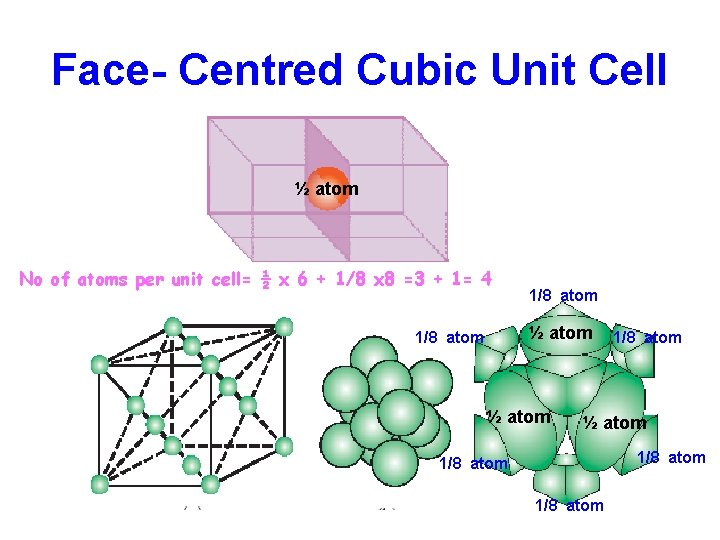Face- Centred Cubic Unit Cell ½ atom No of atoms per unit cell= ½ x 6 + 1/8 x 8 =3 + 1= 4 1/8 atom ½ atom 1/8 atom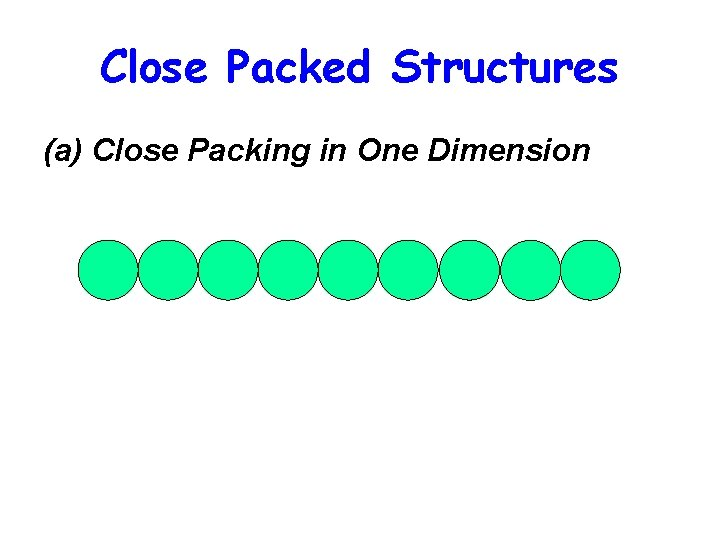Close Packed Structures (a) Close Packing in One Dimension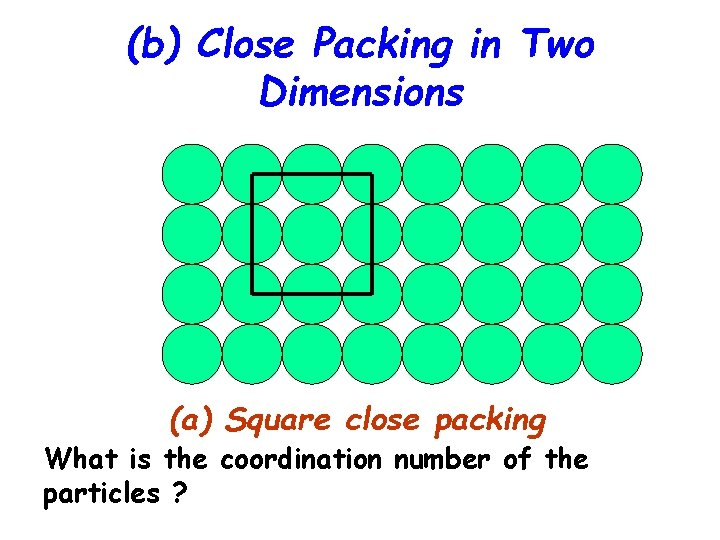(b) Close Packing in Two Dimensions (a) Square close packing What is the coordination number of the particles ?Voids (or holes) Created in Packing of Atoms in a Crystal The free space between spheres in a close packed structure is called ‘Void’ Void or ‘hole’ hole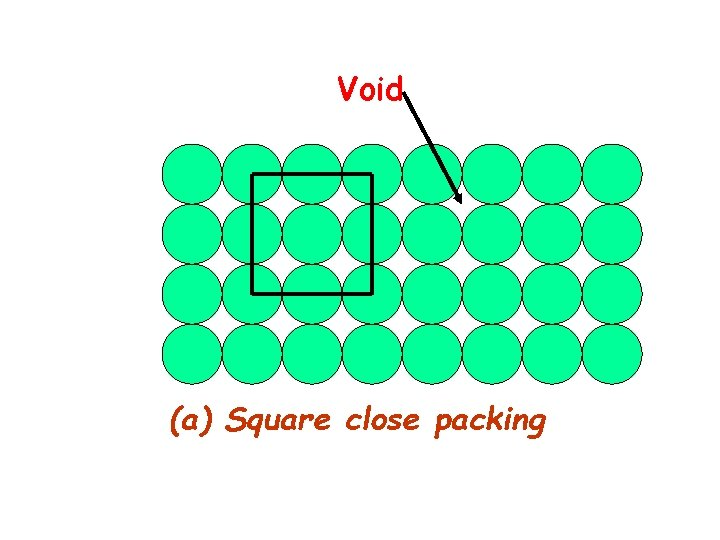Void (a) Square close packing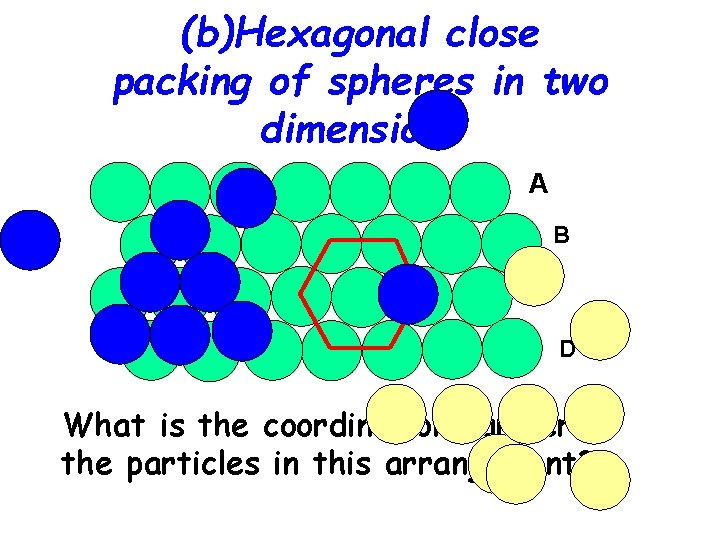(b)Hexagonal close packing of spheres in two dimensions A B C D What is the coordination number of the particles in this arrangement?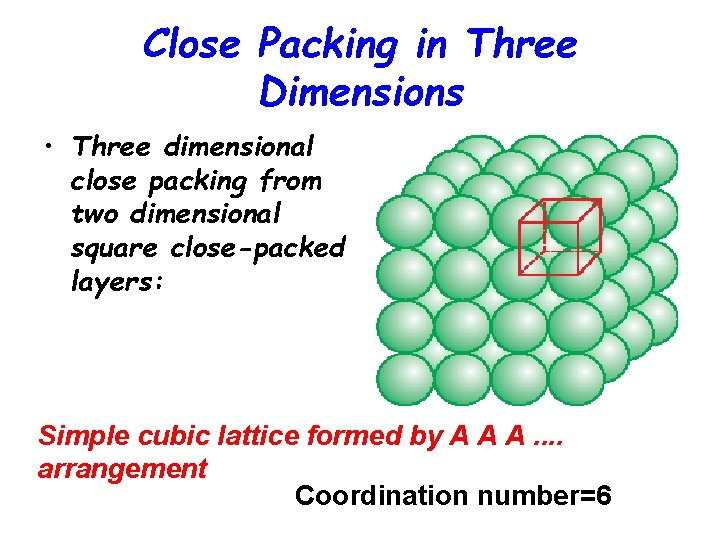Close Packing in Three Dimensions • Three dimensional close packing from two dimensional square close-packed layers: Simple cubic lattice formed by A A A. . arrangement Coordination number=6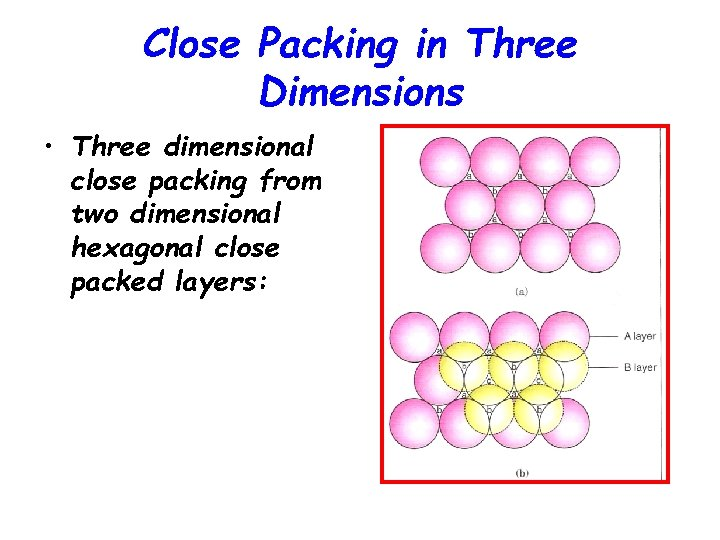Close Packing in Three Dimensions • Three dimensional close packing from two dimensional hexagonal close packed layers: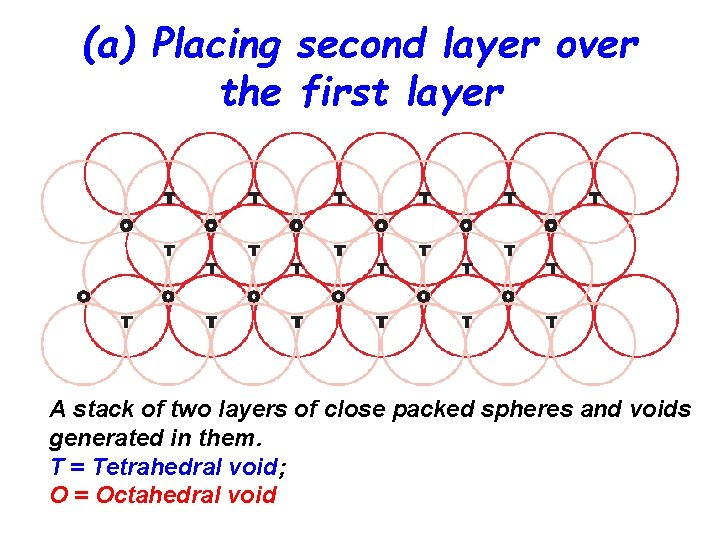(a) Placing second layer over the first layer A stack of two layers of close packed spheres and voids generated in them. T = Tetrahedral void; O = Octahedral void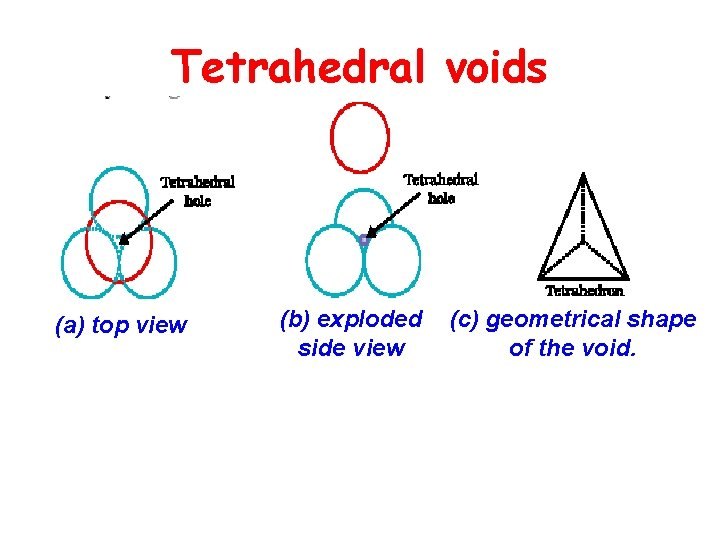Tetrahedral voids (a) top view (b) exploded side view (c) geometrical shape of the void.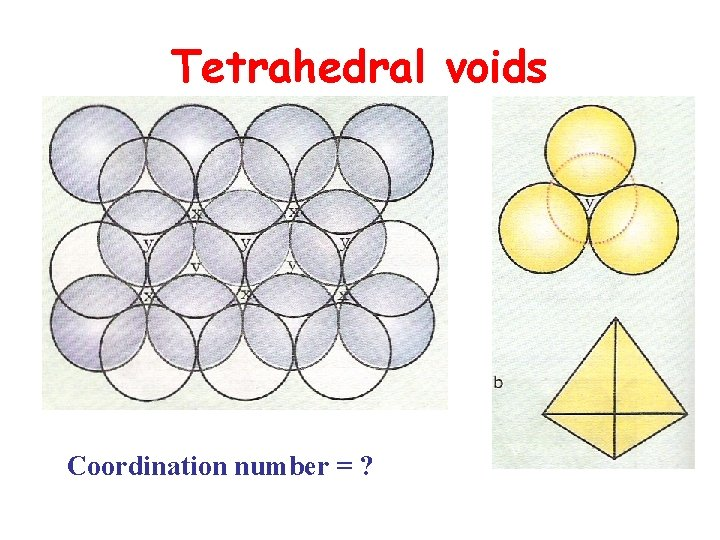Tetrahedral voids Coordination number = ?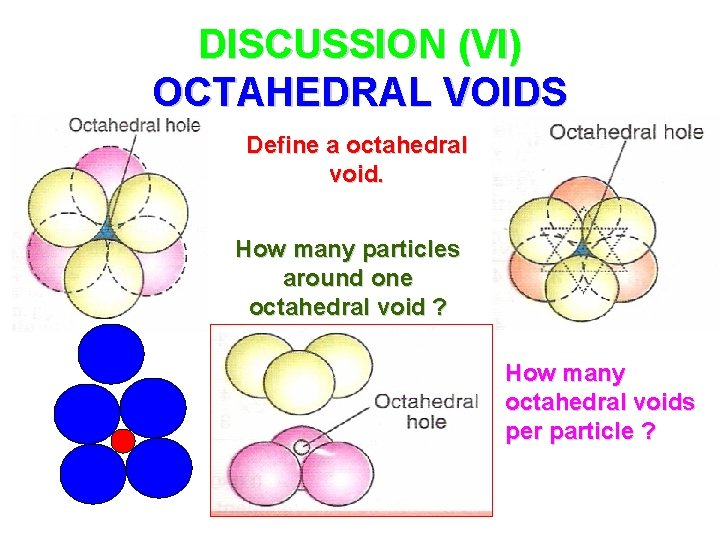DISCUSSION (VI) OCTAHEDRAL VOIDS Define a octahedral void. How many particles around one octahedral void ? How many octahedral voids per particle ?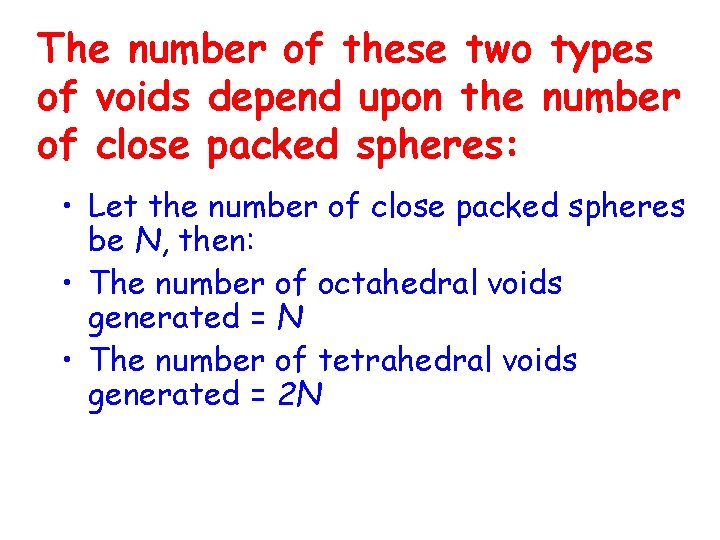The number of these two types of voids depend upon the number of close packed spheres: • Let the number of close packed spheres be N, then: • The number of octahedral voids generated = N • The number of tetrahedral voids generated = 2 N(b) Placing third layer over the second layer When third layer is placed over the second, there are two possibilities. (i) Covering Tetrahedral Voids: (ii) Covering Octahedral Voids:Covering Tetrahedral Voids: (a)Hexagonal cubic close-packing exploded view showing stacking of layers of spheres (b)four layers stacked in each case and (c)geometry of packing. Packing fraction = 74% coordination number = 12Covering Octahedral Voids: a) ABCABC. . arrangement of layers when octahedral void is covered b) Fragment of structure formed by this arrangement resulting in cubic closed packed (ccp) or face centred cubic (fcc) structure. Packing fraction = 74% coordination number = 12Locating Tetrahedral Voids (a) Eight tetrahedral voids per unit cell of ccp structure (b) one tetrahedral void showing the geometry.About Tetrahedral Voids • each small cube has atoms at alternate corners. In all, each small cube has 4 atoms. When joined to each other, they make a regular tetrahedron. • thus, there is one tetrahedral void in each small cube and eight tetrahedral voids in total. Each of the eight small cubes have one void in one unit cell of ccp structure. • ccp structure has 4 atoms per unit cell. • Thus, the number of tetrahedral voids is twice the number of atoms.Locating Octahedral Voids (a)at the body centre of the cube and (b)at the centre of each edge (only one such void is shown).About Octahedral Voids In cubic close packed structure: Octahedral void at the body-centre of the cube = 1 12 octahedral voids located at each edge and shared between four unit cells = 12 x 1/4=3 Total number of octahedral voids = 4 You know that in ccp structure, each unit cell has 4 atoms. Thus, the number of octahedral voids is equal to this number.• It is impossible to pack identical spheres together with a coordination number greater than 12. • The close packed structure adopted by a metal is associated with lowest energy.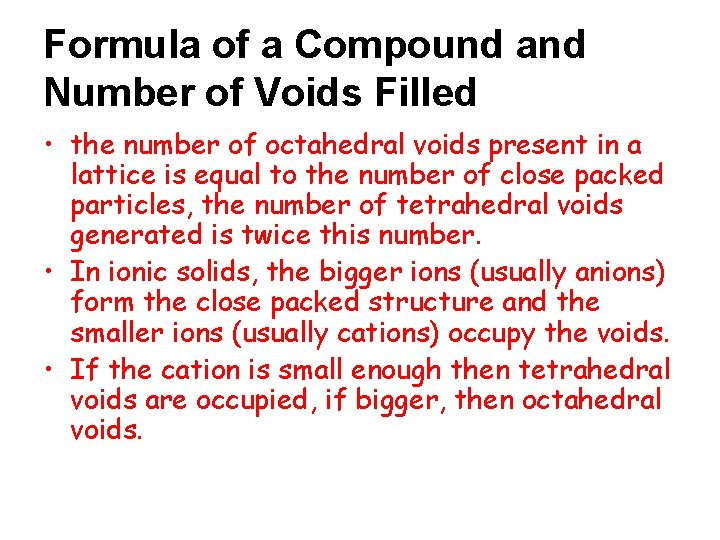Formula of a Compound and Number of Voids Filled • the number of octahedral voids present in a lattice is equal to the number of close packed particles, the number of tetrahedral voids generated is twice this number. • In ionic solids, the bigger ions (usually anions) form the close packed structure and the smaller ions (usually cations) occupy the voids. • If the cation is small enough then tetrahedral voids are occupied, if bigger, then octahedral voids.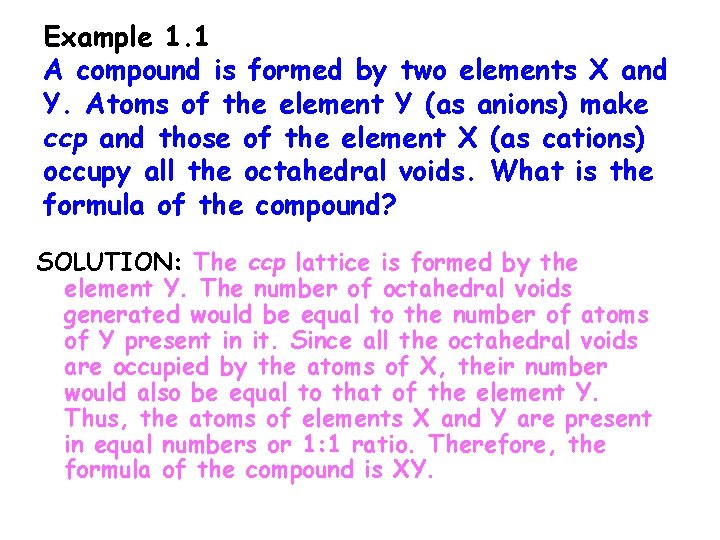Example 1. 1 A compound is formed by two elements X and Y. Atoms of the element Y (as anions) make ccp and those of the element X (as cations) occupy all the octahedral voids. What is the formula of the compound? SOLUTION: The ccp lattice is formed by the element Y. The number of octahedral voids generated would be equal to the number of atoms of Y present in it. Since all the octahedral voids are occupied by the atoms of X, their number would also be equal to that of the element Y. Thus, the atoms of elements X and Y are present in equal numbers or 1: 1 ratio. Therefore, the formula of the compound is XY.Example 1. 2 Atoms of element B form hcp lattice and those of the element A occupy 2/3 rd of tetrahedral voids. What is the formula of the compound formed by the elements A and B? Solution: The number of tetrahedral voids formed is equal to twice the number of atoms of element B and only 2/3 rd of these are occupied by the atoms of element A. Hence the ratio of the number of atoms of A and B is 2 × (2/3): 1 or 4: 3 and the formula of the compound is A 4 B 3.